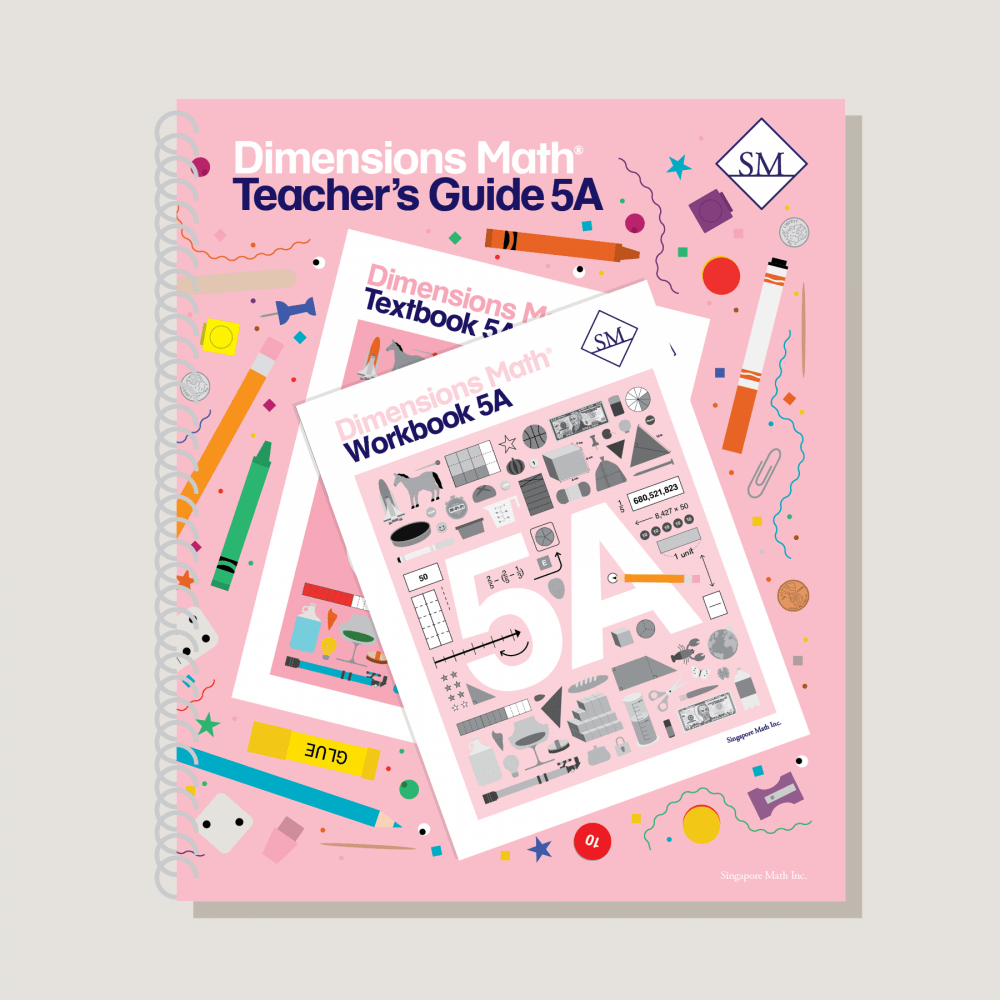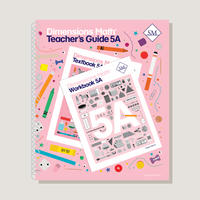# Dimensions Math Teacher's Guide 5A

\$29.50

Teacher’s guides are a comprehensive resource for achieving lesson objectives. They include background information, detailed lesson plans, helpful suggestions, and activities. They help educators understand the purpose of each lesson within the context of the curriculum. A high level of educator involvement is essential for success in this program.

Note: Hard cover. Spiral-bound.

SKU: DMTG5A

ISBN: 9781947226401

Pagecount: 296

Dimensions: 11 x 12 x 1 in

Weight: 2 lbs

Binding: Spiral

Color: Color

Cover: Hard

Perforated: No

Sample Pages

Chapter 1: Whole Numbers
Teaching Notes
Chapter Opener
Lesson 1: Numbers to One Billion
Lesson 2: Multiplying by 10, 100, and 1,000
Lesson 3: Dividing by 10, 100, and 1,000
Lesson 4: Multiplying by Tens, Hundreds, and Thousands
Lesson 5: Dividing by Tens, Hundreds, and Thousands
Lesson 6: Practice
Workbook Solutions

Chapter 2: Writing and Evaluating Expressions
Teaching Notes
Chapter Opener
Lesson 1: Expressions with Parentheses
Lesson 2: Order of Operations — Part 1
Lesson 3: Order of Operations — Part 2
Lesson 4: Other Ways to Write and Evaluate Expressions
Lesson 5: Word Problems — Part 1
Lesson 6: Word Problems — Part 2
Lesson 7: Practice
Workbook Solutions

Chapter 3: Multiplication and Division
Teaching Notes
Chapter Opener
Lesson 1: Multiplying by a 2-digit Number — Part 1
Lesson 2: Multiplying by a 2-digit Number — Part 2
Lesson 3: Practice A
Lesson 4: Dividing by a Multiple of Ten
Lesson 5: Divide a 2-digit Number by a 2-digit Number
Lesson 6: Divide a 3-digit Number by a 2-digit Number — Part 1
Lesson 7: Divide a 3-digit Number by a 2-digit Number — Part 2
Lesson 8: Divide a 4-digit Number by a 2-digit Number
Lesson 9: Practice B
Workbook Solutions

Chapter 4: Addition and Subtraction of Fractions
Teaching Notes
Chapter Opener
Lesson 1: Fractions and Division
Lesson 3: Subtracting Unlike Fractions
Lesson 4: Practice A
Lesson 5: Adding Mixed Numbers— Part 1
Lesson 6: Adding Mixed Numbers— Part 2
Lesson 7: Subtracting Mixed Numbers— Part 1
Lesson 8: Subtracting Mixed Numbers— Part 2
Lesson 9: Practice B
Workbook Solutions

Chapter 5: Multiplication of Fractions
Teaching Notes
Chapter Opener
Lesson 1: Multiplying a Fraction by a Whole Number
Lesson 2: Multiplying a Whole Number by a Fraction
Lesson 3: Word Problems — Part 1
Lesson 4: Practice A
Lesson 5: Multiplying a Fraction by a Unit Fraction
Lesson 6: Multiplying a Fraction by a Fraction — Part 1
Lesson 7: Multiplying a Fraction by a Fraction — Part 2
Lesson 8: Multiplying Mixed Numbers
Lesson 9: Word Problems — Part 2
Lesson 10: Fractions and Reciprocals
Lesson 11: Practice B
Review 1
Workbook Solutions

Chapter 6: Division of Fractions
Teaching Notes
Chapter Opener
Lesson 1: Dividing a Unit Fraction by a Whole Number
Lesson 2: Dividing a Fraction by a Whole Number
Lesson 3: Practice A
Lesson 4: Dividing a Whole Number by a Unit Fraction
Lesson 5: Dividing a Whole Number by a Fraction
Lesson 6: Word Problems
Lesson 7: Practice B
Workbook Solutions

Chapter 7: Measurement
Teaching Notes
Chapter Opener
Lesson 1: Fractions and Measurement Conversions
Lesson 2: Fractions and Area
Lesson 3: Practice A
Lesson 4: Area of a Triangle — Part 1
Lesson 5: Area of a Triangle — Part 2
Lesson 6: Area of Complex Figures
Lesson 7: Practice B
Workbook Solutions

Chapter 8: Volume of Solid Figures
Teaching Notes
Chapter Opener
Lesson 1: Cubic Units
Lesson 2: Volume of Cuboids
Lesson 3: Finding the Length of an Edge
Lesson 4: Practice A
Lesson 5: Volume of Complex Shapes
Lesson 6: Volume and Capacity — Part 1
Lesson 7: Volume and Capacity — Part 2
Lesson 8: Practice B
Review 2
Workbook Solutions

A & B Books: Our programs divide the school year into two semesters. “A” level books are for the first half of the school year. “B” level books are for the second half of the school year. You need both “A” and “B” material for a complete school year.

Required Components: Textbooks, Workbooks, and Guides (either Home Instructor’s Guides or Teacher’s Guides) are all necessary components. These three elements each serve a unique function and work together to build math mastery.

Printouts: Dimensions Math PK-5 Resources

Recommended Manipulatives: Dimensions Math Grades PK-5 Recommended Manipulatives